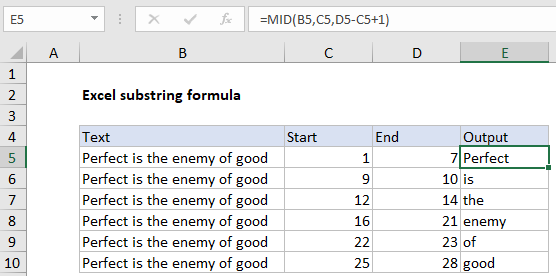## Excel Office

Excel How Tos, Tutorials, Tips & Tricks, Shortcuts

# How to extract substring in Excel

To extract a substring with an Excel formula, you can use the MID function.

Note: In this example, we are calculating the end position in order to extract a substring with a literal start and end position. However, if you know the number of characters to extract, you can just plug in that number directly.

Worked Example:   How to convert text string to array in Excel

## Formula

`=MID(A1,start,end-start+1)`## Explanation

In the example shown, the formula in E5 is:

`=MID(B5,C5,D5-C5+1)`

which, on row 5, returns “Perfect”.

### How this formula works

In the example on this page, we are using the MID function to extract text based on a start and end position. The MID function accepts three arguments: a text string, a starting position, and the number of characters to extract. The text comes from column B, and the starting position comes from column C. The number of characters to extract is calculated by subtracting the start from end, and adding 1. In cell E6:

```=MID(B5,C5,D5-C5+1)
=MID("Perfect is the enemy of good",1,7-1+1)
=MID(B5,1,7) // returns "Perfect"```

### Functions to extract substrings

Excel provides three primary functions for extracting substrings:

```=MID(txt,start,chars) // extract from middle
=LEFT(txt,chars) // extract from left
=RIGHT(txt,chars) // extract from right```

### Finding start and end positions with a formula

In the example shown, start and end positions are based on hardcoded values. However, it is possible to calculate positions with the FIND function and SEARCH function.

Worked Example:   How to check if cell contains all of many things in Excel
Worked Example:   How to check cell that contains one of many with exclusions in Excel